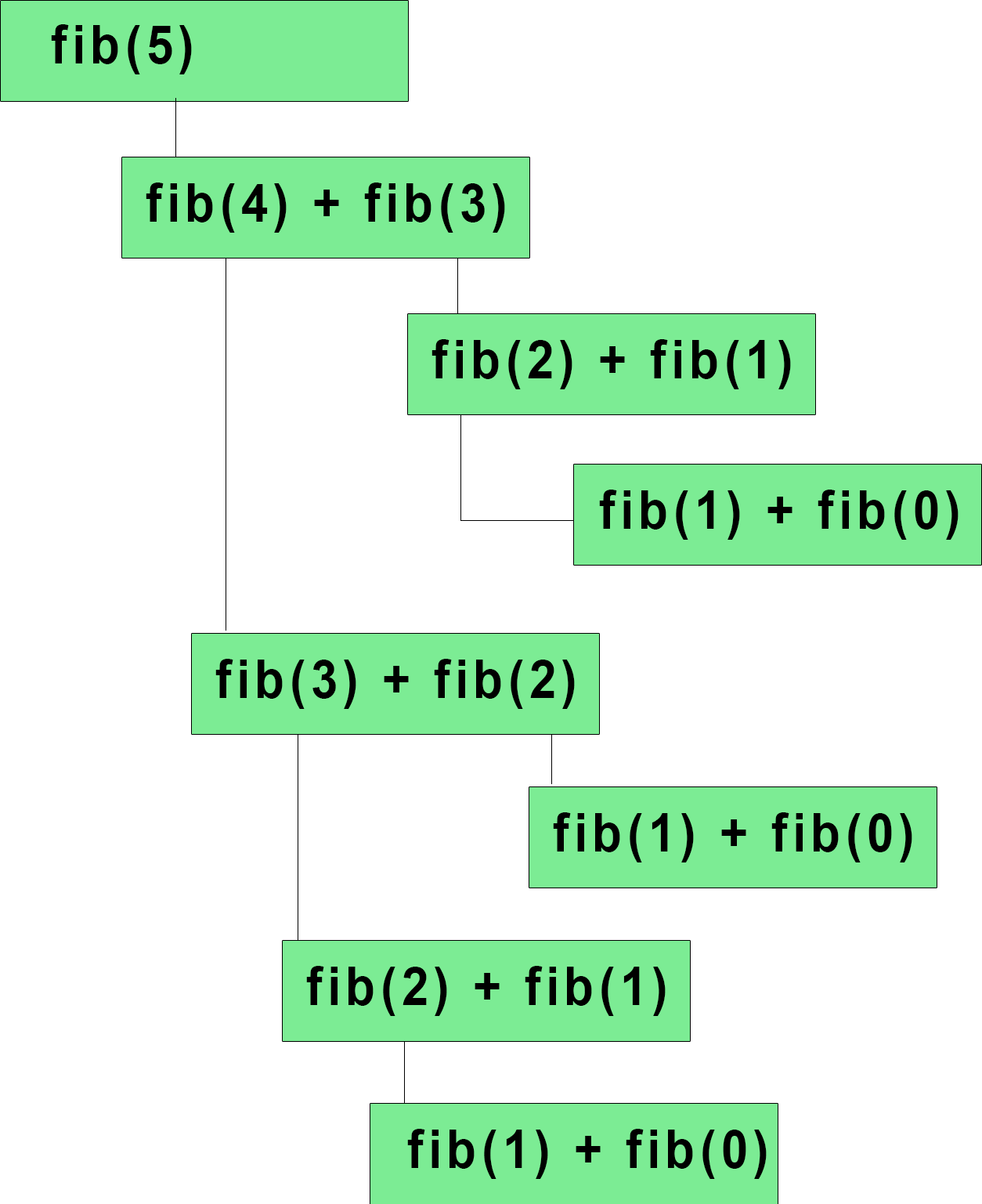# When not to use recursion

Aug. 8, 2017 21164

With no doubt, recursion is a great tool and is a natural way to express many algorithms in an easily comprehensible way. But every good thing comes with a cost, and this post is about the pros and cons of using recursion, telling you when you should use it and when you shouldn't.

Let's first discuss the steps performed when a function is called:

1. Space is made on the stack for the function's arguments and local variables
2.  Function's arguments are copied into this space
3.  Function's code executes
4.  Stack goes to its previous position

Doing all of these takes a little bit more time than iterating through a loop but the real problem with recursion is the first step. Every time a recursive call is made, a stack space is allocated to store the local variables and because of this, the program may cause stack overflow problem if the recursive call is large in number.

Let's write a recursive function to calculate the factorial of a number.

fac(int n)
if (n == 0 OR n == 1)
return 1
else
return a*fac(a-1)


Calculating 1000! with this program will use 1000 stack frames.

fac(int n)
i = 1;
while n>1
i = i*n
n = n-1
return


But the iterative solution will mainly use just one stack frame.

Let's take an example of calculating the Fibonacci series.

fib(int n)
if (n == 0 OR n == 1)
return 1
else
return fib(n-1)+fib(n-2)


This is the natural way of writing the Fibonacci series because according to the definition a Fibonacci number is 1 if 'n' is 0 or 1. Else it is f(n-1)+f(n-2) and the above code represents this in the most natural way. But the major problem with this is that the same value will be calculated again and again for calculation of a Fibonacci term and this will take more time and more importantly, more stack space. For example, let's calculate fib(5) with the above-given algorithm.You can see that fib(5) leads to the calculation of fib(4) and fib(3). And fib(4) leads to the calculation of fib(3) and fib(2). So, till this step, only fib(3) is calculated twice. Similarly, fib(2) is calculated multiple times in this example.

So even though recursion represented the algorithm in a natural way, it is very inefficient in this case.

Thus, recursion may cause memory overflow if your stack space is large, and is also inefficient in cases where the same value is calculated again and again. So, use either iteration or recursion according to the task you want to perform. If simplicity is required and memory overflow is not a major issue then use recursion otherwise go with iterations.

Liked the post?
Developer and founder of CodesDope.
0 COMMENT